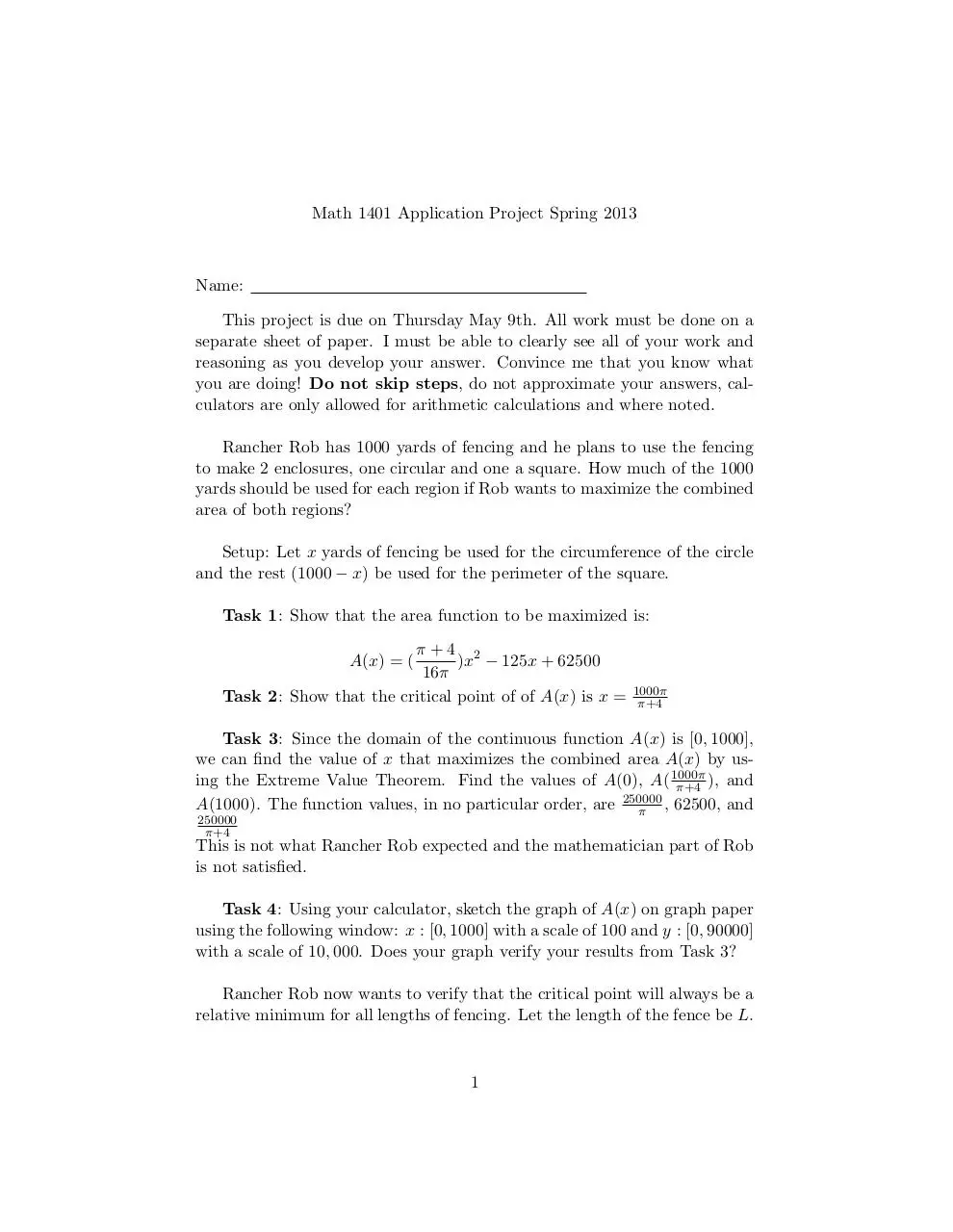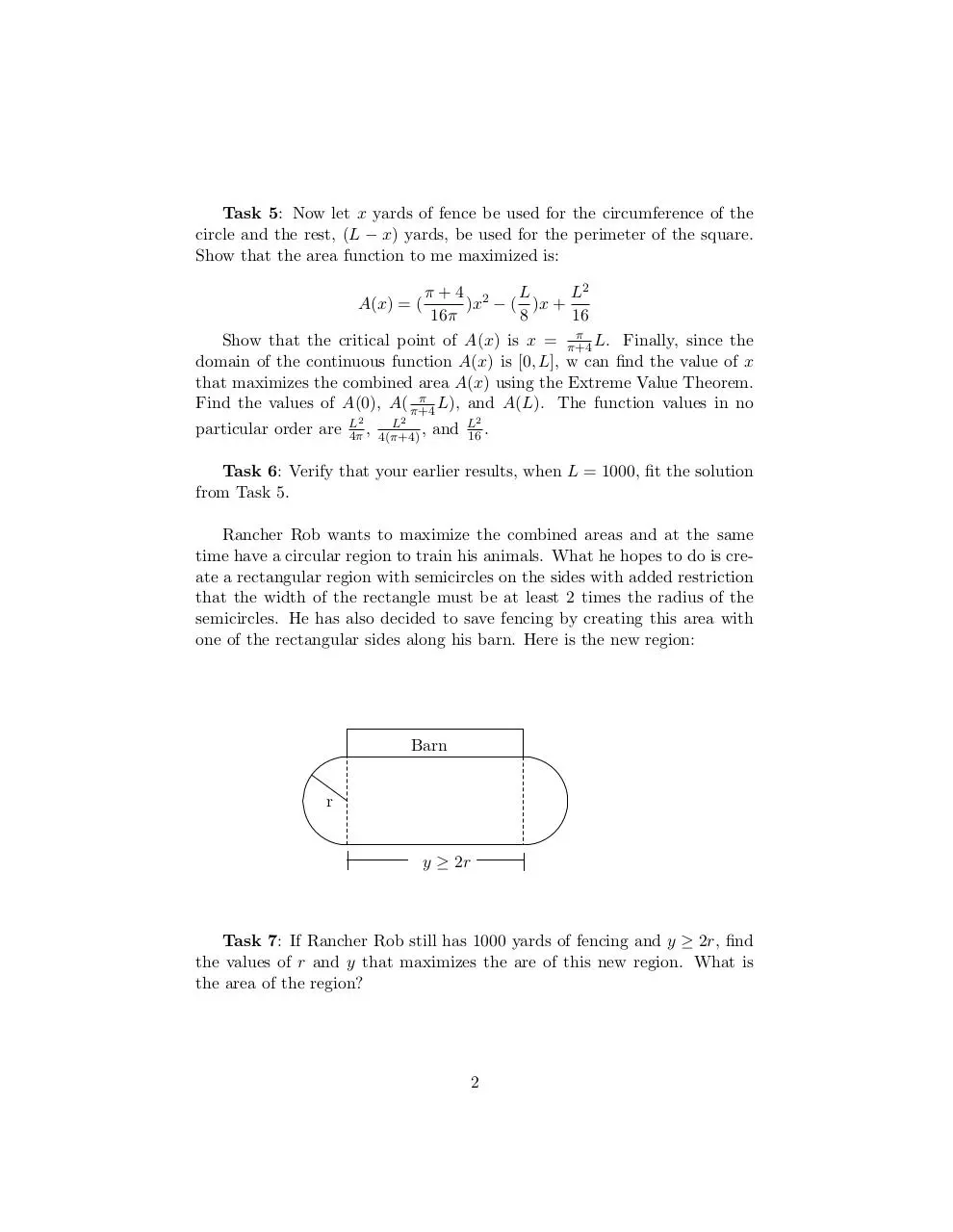# Application Project .pdf

### File information

This PDF 1.5 document has been generated by TeX / MiKTeX pdfTeX-1.40.12, and has been sent on pdf-archive.com on 01/05/2013 at 12:39, from IP address 75.171.x.x. The current document download page has been viewed 1181 times.
File size: 84.42 KB (2 pages).
Privacy: public file

### Document preview

Math 1401 Application Project Spring 2013

Name:
This project is due on Thursday May 9th. All work must be done on a
separate sheet of paper. I must be able to clearly see all of your work and
reasoning as you develop your answer. Convince me that you know what
you are doing! Do not skip steps, do not approximate your answers, calculators are only allowed for arithmetic calculations and where noted.
Rancher Rob has 1000 yards of fencing and he plans to use the fencing
to make 2 enclosures, one circular and one a square. How much of the 1000
yards should be used for each region if Rob wants to maximize the combined
area of both regions?
Setup: Let x yards of fencing be used for the circumference of the circle
and the rest (1000 − x) be used for the perimeter of the square.
Task 1: Show that the area function to be maximized is:
π+4 2
)x − 125x + 62500
16π
Task 2: Show that the critical point of of A(x) is x =
A(x) = (

1000π
π+4

Task 3: Since the domain of the continuous function A(x) is [0, 1000],
we can find the value of x that maximizes the combined area A(x) by using the Extreme Value Theorem. Find the values of A(0), A( 1000π
π+4 ), and
250000
A(1000). The function values, in no particular order, are π , 62500, and
250000
π+4

This is not what Rancher Rob expected and the mathematician part of Rob
is not satisfied.
Task 4: Using your calculator, sketch the graph of A(x) on graph paper
using the following window: x : [0, 1000] with a scale of 100 and y : [0, 90000]
Rancher Rob now wants to verify that the critical point will always be a
relative minimum for all lengths of fencing. Let the length of the fence be L.

1

Task 5: Now let x yards of fence be used for the circumference of the
circle and the rest, (L − x) yards, be used for the perimeter of the square.
Show that the area function to me maximized is:
π+4 2
L
L2
)x − ( )x +
16π
8
16
π
Show that the critical point of A(x) is x = π+4
L. Finally, since the
domain of the continuous function A(x) is [0, L], w can find the value of x
that maximizes the combined area A(x) using the Extreme Value Theorem.
π
Find the values of A(0), A( π+4
L), and A(L). The function values in no
A(x) = (

particular order are

L2
L2
4π , 4(π+4) ,

and

L2
16 .

Task 6: Verify that your earlier results, when L = 1000, fit the solution
Rancher Rob wants to maximize the combined areas and at the same
time have a circular region to train his animals. What he hopes to do is create a rectangular region with semicircles on the sides with added restriction
that the width of the rectangle must be at least 2 times the radius of the
semicircles. He has also decided to save fencing by creating this area with
one of the rectangular sides along his barn. Here is the new region:

Barn
r

y ≥ 2r

Task 7: If Rancher Rob still has 1000 yards of fencing and y ≥ 2r, find
the values of r and y that maximizes the are of this new region. What is
the area of the region?

2Application Project.pdf (PDF, 84.42 KB)

### Share on social networks

#### HTML Code

Copy the following HTML code to share your document on a Website or Blog